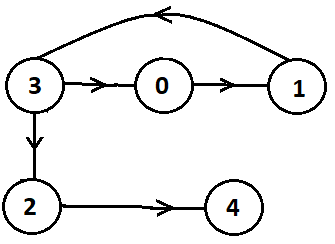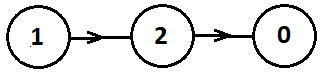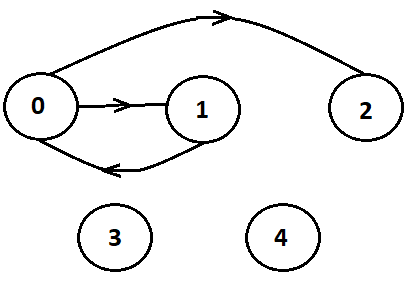Problem title
Difficulty
Avg time to solve

Path With Minimum Effort
Hard
20 mins
Count Of Range Sum
Moderate
20 mins
Stones falling
Hard
45 mins
Safe Nodes In The Graph
Moderate
15 mins
Graph Valid Tree
Moderate
30 mins
Similar String Groups
Moderate
35 mins
Most Lucky String
Hard
45 mins
NINJA AND HIS GIRLFRIEND
Moderate
25 mins
Ninja visits his friend
Moderate
30 mins
Toggle bulb’s switches
Easy
15 mins

# Safe Nodes In The Graph

Difficulty: MEDIUM
Avg. time to solve
15 min
Success Rate
85%

Problem Statement

#### For Example: For the graph, as shown in the picture below, [2, 4] are the only safe nodes.#### Ninja wants to know all the safe nodes in the graph in sorted order. Can you help Ninja to find out all the safe nodes?

##### Input Format
``````The first line of input contains an integer 'T' representing the number of test cases. Then the test cases follow.

The first line of each test case contains an integer ‘N’ and ‘E’ representing the number of nodes and edges in the graph.

The next ‘E’ lines of each test case contain two single space-separated integers denoting ‘EDGES[i]’ and ‘EDGES[i]’ which is a directed edge from node ‘EDGES[i]’ to ‘EDGE[i]’.
``````
##### Output Format :
``````For each test case, print the safe nodes in sorted order.

The output for each test case is printed in a separate line.
``````

#### Note:

``````You do not need to print anything; it has already been taken care of. Just implement the given function.
``````
##### Constraints :
``````1 <= T <= 100
1 <= N <= 10 ^ 4
1 <= E <= 10 ^ 4
0 <= EDGE[i] and EDGE[i] < N

Where ‘EDGE[i]’ and ‘EDGE[i]’ represents the directed edge.

Time Limit: 1 sec
``````
##### Sample Input 1 :
``````2
3 2
1 2
2 0
2 0
``````
##### Sample Output 1:
``````0 1 2
0 1
``````

#### Explanation for Sample Output 1:

``````For the first test case:
[0, 1, 2] are the safe nodes. See the picture below for your reference:
````````````For the second case:
[0, 1] are the safe nodes. Because there is no edge between nodes so each node is a starting and an ending node.
``````
##### Sample Input 2 :
``````2
5 3
0 1
1 0
0 2
2 2
0 1
1 0
``````
##### Sample Output 2 :
``````2 3 4
``````

#### Explanation for Sample Output 1:

``````For the first test case:
[2, 3, 4] are the safe nodes. See the picture below for your reference:
````````````For the second test case:
There are no safe nodes. So we return an empty array/list.
``````Console